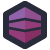So much bad date-handling code is based in people reinventing methods that already exist, badly. That's code that solves a problem people have, that's already solved. But the really special code solves a problem nobody understands or wants solved.

Take this C# function from Luke's co-worker.

``````        /// <summary>
/// Gets the start date by two dates and no of week.
/// </summary>
/// <param name="dtFrom">The dt from.</param>
/// <param name="dtTo">The dt to.</param>
/// <param name="month">The month.</param>
/// <returns></returns>
private DateTime GetStartDateByTwoDatesAndNoOfWeek(DateTime dtFrom, DateTime dtTo, int month)
{
DateTime dtReturn = dtFrom;

// Calculate the total number of weeks by given from and to date.
TimeSpan tt = dtTo - dtFrom;
int totalWeeks = tt.Days / 7;

// Calculate the number of weeks by given number of month
int noOfWeek = month * 4;

// If the total number of weeks between the two dates is equal to the number of weeks
// calculate by the given number of month then return given from date otherwise
// start adding or subtracting one week from the date.
if (totalWeeks.Equals(noOfWeek))
dtReturn = dtFrom;
else if (noOfWeek < totalWeeks)
{
for (int i = totalWeeks; i > noOfWeek; i--)
{
}
}
else // noOfWeek > totalWeeks
{
for (int i = totalWeeks; i < noOfWeek; i++)
{
}
}

return dtReturn;
}
``````

So, this function "gets start date", from "two dates" and a "number of weeks". The comment tells me this, the method name tells me this, and the function parameters tell me that it actually takes a number of months, not weeks.

But let's trace through the code.

We start by setting our return value to `dtFrom`. Then we count how many weeks are between our `dtTo` and `dtFrom`. Then we take the month input and convert it into weeks, by simply multiplying by 4. That's… not accurate. I mean, it's the floor of the number of weeks per month, so maybe it's fine.

If the number of weeks between the origin dates is the same as the one calculated by months, we set the return value back to `dtFrom`, which is what we just set it to anyway. But if the number of weeks mismatches, we add or subtract days. Mind you, we add or subtract in a loop, instead of just doing some basic arithmetic.

Then it returns the resulting date. Why? To what end? What is this for?[Advertisement] Continuously monitor your servers for configuration changes, and report when there's configuration drift. Get started with Otter today!